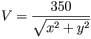# Electric Potential in a region of space

## Homework Statement

The electric potential in a region of space is, where x and y are in meters. What is the strength of the electric field at (x,y)=(2.1m,2.7m)?
The electric force is in V/m.

E = -dV/ds

## The Attempt at a Solution

I know that i have to find the partial derivatives of the x and the y but im not sure on how to get the answer from the equation given. I was thinking of making the square root into the exponential of 1/2 and then raising that to -1 to get ride of the fraction and then taking the derivatives of that but i keep getting the wrong answer. Anybody have suggestions of help they can give me please. Thanks.

tiny-tim
Homework Helper
Welcome to PF!…I know that i have to find the partial derivatives of the x and the y but im not sure on how to get the answer from the equation given. I was thinking of making the square root into the exponential of 1/2 and then raising that to -1 to get ride of the fraction and then taking the derivatives of that but i keep getting the wrong answer. Anybody have suggestions of help they can give me please. Thanks.

Hi quietriot1006! Welcome to PF!(btw, please use the LaTeX provided, rather than importing images of equations)

Just use the Chain Rule for differentiation …

you know how to differentiate 1/√x, don't you?Thanks for the welcome. Im still not getting the answer. Maybe im doing the chain rule incorrectly. What would i use for u or g(x) and h(x)?

tiny-tim
Homework Helper
Thanks for the welcome. Im still not getting the answer. Maybe im doing the chain rule incorrectly. What would i use for u or g(x) and h(x)?

(x2 + y2)I keep getting the same asnwer, -127 V/M and its not the right answer. What else can i do?

tiny-tim
Show us your calculations, and then we can see where the mistake is.